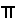### The Animated Tangent and Secant

Tangent and Cotangent Trig Tables

The Sine and Cosine
 The triangle touching the unit circle at points (x,y) determines the sine and cosine functions.The triangle has: a vertical leg, THE SINE FUNCTION, with endpoints at (x,y) - a point on the circle - and (x,0) - a point on the x-axis, a horizontal leg, THE COSINE FUNCTION, with end points at (x,0) and the origin, (0,0), and a hypotenuse, length (or radius) of 1, with endpoints at the origin and the point (x,y) on the circle.     For detailed information on the sine and cosine functions, go to Function and Relation Library: Trigonometric Functions - sine or cosine.Zero Degrees, Zero Radians, ZeroFirst Quadrant90 Degrees,/2 Radians,Second Quadrant180 Degrees,Radians,Third Quadrant270 Degrees, 3/2 Radians, 3/2Fourth Quadrant© 2007, Agnes Azzolino www.mathnstuff.com/math/spoken/here/2class/330/unittan.htm